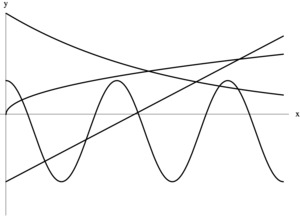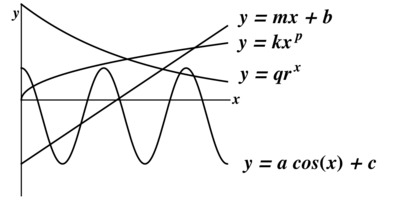# Analyzing Graphs

Alignments to Content Standards: F-IF.C

Pictured below are the graphs of four different functions, defined in terms of eight constants: $a, b, c, k, m, p, q, \text{ and } r.$ The equations of the functions are:

• $y=mx+b$
• $y=a\cos(x)+c$
• $y=qr^x$
• $y=kx^p$1. Match each equation with its graph.
1. Which of the 8 constants are definitely negative?
2. Which of the 8 constants are definitely positive?
3. Which of the 8 constants are definitely greater than zero but less than one?
4. Which of the 8 constants are definitely greater than one?

## IM Commentary

The task could be used as a review problem or as an assessment problem after many different types of functions have been discussed. Since the different parameters of the functions are not given explicitly, the focus is not just on graphing specific functions but rather students have to focus on how values of parameters are reflected in a graph. How is the amplitude of a sinusoidal function reflected in the graph? What can we say about the exponent of a power function if the function is concave down?

This task lends itself to have students construct viable arguments (SMP 3). Rather than using memorization to find the answers, students should reason why a constant has to be positive or negative.

A teacher could also choose to let their students use a graphing calculator to graph functions with different values of the constant to form conjectures. Then they could explain why their conjectures are true in general.

Task based on a problem by Jerry Morris, Sonoma State University. Used with permission.

## Solution

1. From the form of the equations, we can see that $y=mx+b$ is a linear function, $y=kx^p$ is a power function, $y=qr^x$ is an exponential function, and $y=a\cos x + c$ is a trigonometric function. Knowing the general shape of these functions or using a graphing calculator to plot specific functions of each type, we find the following matching:1. The constant $b$ is definitely negative since the line shown in the graph intersects the negative portion of the $y$-axis, meaning that the $y$-intercept is negative. The constant $c$ is also definitely negative because it represents the midline of the trigonometric function that is shown, and we can see that the midline of this function lies below the $x$-axis. To summarize, our answers are as follows:

$$\text{Constants that are definitely negative:}\ b \text{ and } c$$

2. The constant $k$ is definitely positive because the graph of the power function $y=kx^p$ lies above the $x$-axis. Similary, the constant $q$ is definitely positive because the exponential function shown lies above the $x$-axis. The constant $m$ is definitely positive because it represents the slope of the line that is shown, and we can see that the line rises from left to right. The constant $p$ is definitely positive because the graph of the power function is increasing. The constant $r$ is definitely positive because exponential functions must always have positive bases, by definition. Finally, the constant $a$ is definitely positive since the cosine function starts at its maximum value. To summarize, our answers are as follows: $$\text{Constants that are definitely positive:}\ k,\ q,\ m,\ p,\ r \text{ and } a$$
3. The constant $p$ is definitely greater than zero but less than one because the power function is concave downward (like the graph of $y=x^{1/2},$ for example.) The constant $r$ is definitely greater than zero but less than one because the exponential function $y = qr^x$ is decreasing, implying that its base must be less than one. To summarize, our answers are as follows: $$\text{Constants that are definitely greater than 0 but less than 1:}\ p \text{ and } r$$
4. We cannot say for sure that any of the constants are greater than one. Therefore, none of the constants are definitely greater than one.